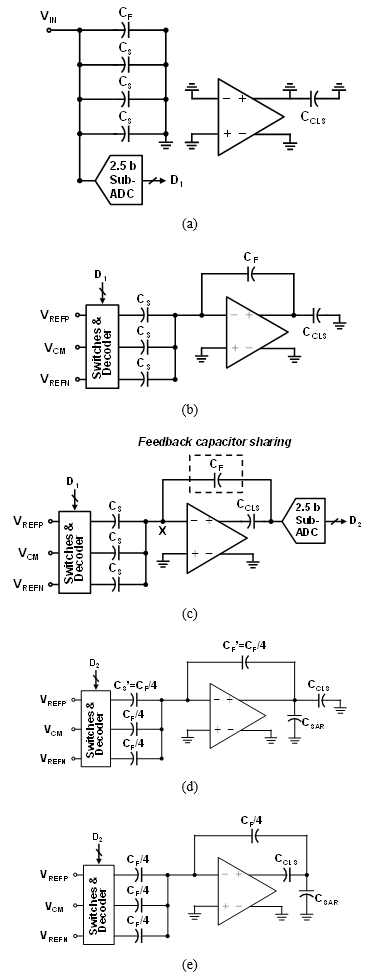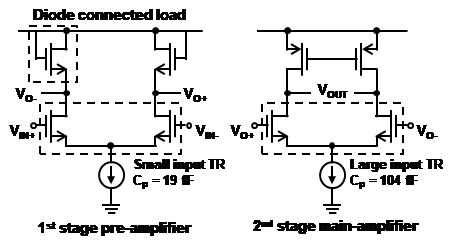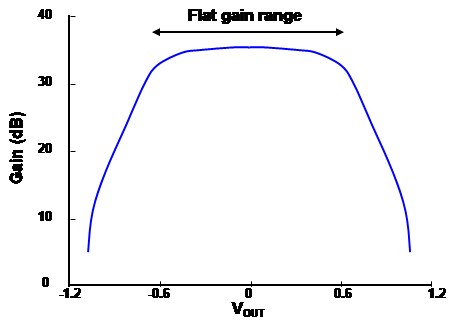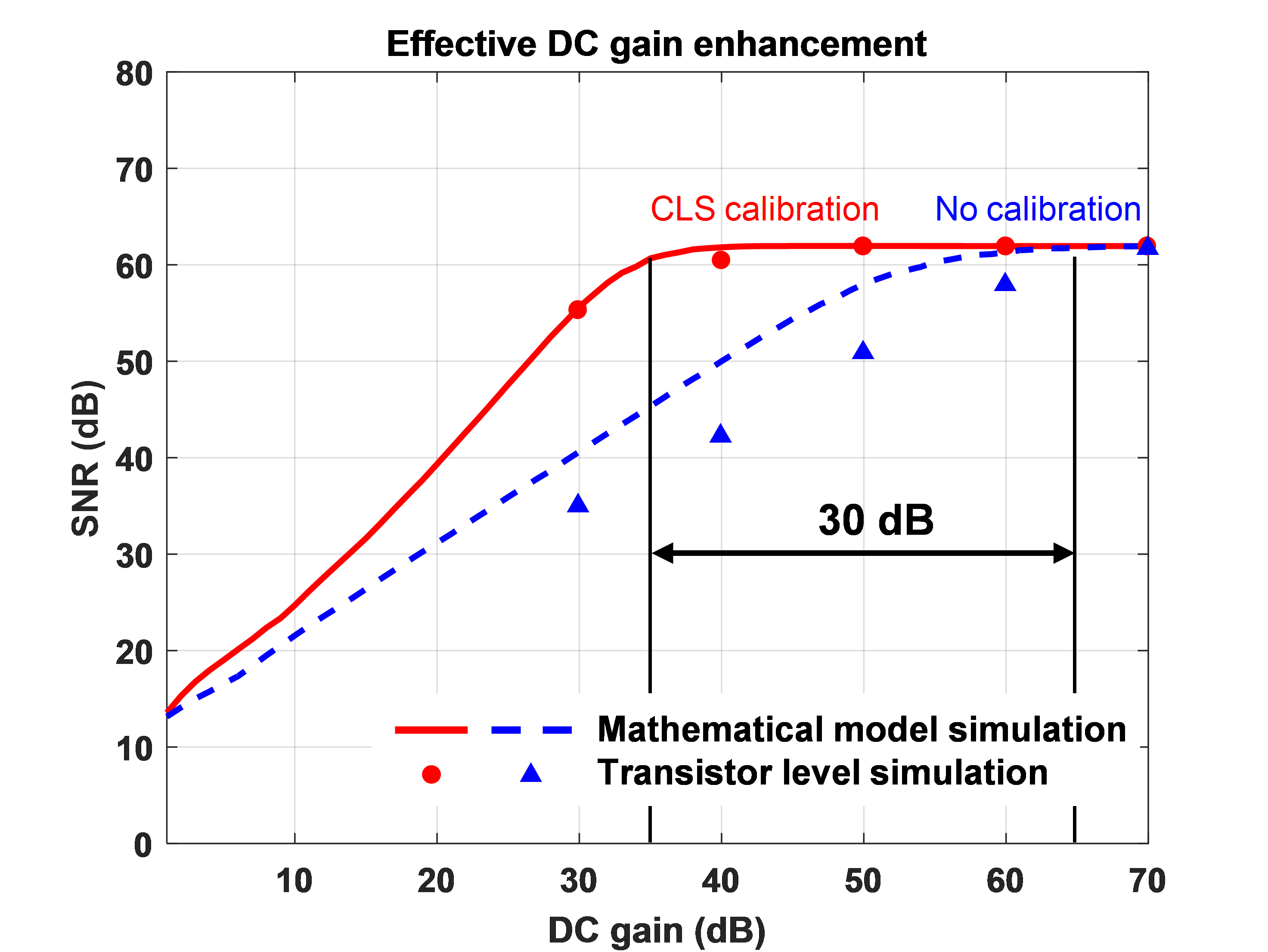Mobile QR Code## I. Introduction

Low-power analog to digital converters (ADCs) are important building blocks for portable applications such as wireless communication systems, imaging, and video signal processing. ADCs with a medium resolution (8-10 bits) and tens of million samples per second (MS/s) in sampling frequencies are required for power efficient portable systems. Among Nyquist-rate ADC architectures, both pipeline and successive approximation register (SAR) ADCs are good candidates for those systems.

A SAR ADC consisting of a comparator, capacitors, switches, and control logics can achieve high power efficiency (1-3) because it does not require an active circuit like an op-amp, which consumes static power. However, conversion speed is limited due to a slow bit-decision process based on a binary search algorithm.

The pipeline ADCs are well known for achieving high resolution and conversion speeds (4-6), but op-amps having large direct current (DC) gains and bandwidth are required. Moreover, it’s difficult to achieve the requirement with modern complementary metal-oxide semiconductor (CMOS) technology when supply voltage has been decreased, and power consumption of the op-amps in nanometer-scale must be increased to fulfill the purpose.

In (7), the first multiplying digital-to-analog (MDAC) stage, which used a SAR ADC as a sub-ADC, 6-bits were generated, while the second stage converted 7-bits. However, the output swing of the MDAC is limited to a quarter of the peak-to-peak voltage (Vpp) because a large DC gain was required from the op-amp, and an input signal amplitude in the second stage had to be reduced. Additionally, the SAR ADC was adopted as a sub-ADC of the MDAC instead of a flash type, so the conversion speed was not impressive. In (8), a large DC gain in the op-amp was necessary, so power consumption was high, while a 9-bit SAR ADC, which has a large chip area, was implemented in the second stage.

In this paper, two techniques were implemented to address the issues of power consumption and DC gain of the op-amp, which have been noted in previous studies. A correlated level shifting (CLS), which is a gain error calibration technique, can reduce the DC gain of the op-amp without losing the signal-to-noise ratio (SNR) performance of the ADC (9). Additionally, a capacitor sharing technique can also decrease the op-amp requirement by reducing the load capacitance by half (10). With both techniques, the power consumption of the op-amp is reduced because the required performance is relaxed.

Section II shows the architecture of this work. Section III shows the circuit description. Sections IV and V show the measurement results and conclusion.

## II. Architecture

Fig. 1 shows a block diagram of the proposed cyclic and SAR combined two-stage ADC. This architecture consists of a cyclic ADC with a CLS gain error calibration and a capacitor sharing in the first stage, and an asynchronous SAR ADC using a binary weighted capacitive DAC in the second stage. The cyclic ADC converting the most significant bit (MSB) generates 5-bits per 2-cycles, while the SAR ADC dealing with the least significant bit (LSB) generates 6-bits. The total 10-bit digital output is then merged using a digital correction method. A benefit of using the cyclic ADC in the first stage is that only a single op-amp is required for the MSB conversion and MDAC operation so power consumption is reduced compared to the pipeline ADC. Moreover, the mitigated requirement of the op-amp compared to the two-stage SAR ADC in (8) is another benefit of the proposed architecture.

Fig. 2 shows a clock timing diagram of the ADC. While the cyclic ADC processes the MSB of the N$^{th}$ sampled input, the SAR ADC processes the LSB of the N-1$^{th}$ sampled signal. Both ADCs operate simultaneously so the conversion speed is increased compared to conventional ADCs. Five clock phases are required to operate two cycles in the 2.5-bit cyclic ADC. The proposed ADC generates the five clocks with internal synchronous logics by receiving 250 MHz external clock. Therefore, the sampling frequency of ADC is determined to 50 MHz.

### 2. Cyclic ADC with CLS Gain-error Calibration in the First Stage

The cyclic ADC used in the first stage is based on a 2.5-bit MDAC structure. Fig. 3 shows an ideal transfer curve for the 2.5-bit MDAC circuit. The sub-ADC used in the MDAC is a flash type, which includes six comparators and generates a digital output of seven levels. An ideal closed loop gain for the 2.5-bit MDAC is four, and is realized with a ratio of capacitors. However, in an actual implementation, the closed loop gain cannot be exactly 4 because the DC gain of the op-amp is finite. In general, op-amps are designed to achieve a large DC gain (more than 70 dB) to guarantee the SNR performance of ADCs.

Fig. 4. Operation of the first cyclic ADC with gain error correction (a) Sampling, (b) 1st estimation, (c) 1st level shift, (d) 2nd estimation, (e) 2nd level shift.However, it is difficult to achieve this large DC gain in op-amps, because the supply voltage of modern CMOS technology has decreased to approximately 1 V for analog circuit designs. If a large DC gain is required and/or a complicated circuit design is involved, the output swing is limited, and power consumption is increased.

In this paper, we adopted a CLS technique, which is a gain error calibration, to the op-amp of the MDAC to reduce the required DC gain and power consumption.

Fig. 4 explains operation of the 2.5-bit MDAC step by step. For simplicity, only a single-ended version is represented, although the implementation is fully differential. In Fig. 4(a), the input signal is directed to sampling capacitors, which are divided into four units. When the sampling is done, a sub-ADC converts the input into a 2.5-bit digital code, which is applied to the sub-DAC, and the op-amp and CLS capacitor ($C_{CLS}$) are reset. The total sampled charge is given by:

##### (1)
$Q_{s}=\left(3 C_{s}+C_{F}\right) \cdot V_{I V}$

where $C_S$ is sampling capacitor, $C_F$ is feedback capacitor, VIN is input voltage, and QS is sampled charge.

In Fig. 4(b), three reference voltages ($V_{REFP}$, VCM, $V_{REFN}$) are connected to the bottom plates of the sampling capacitors depending on the output result of the sub-ADC, $D_{1}$, and the MDAC output is sampled to the $C_{CLS}$, including the gain error of the op-amp. Estimation voltage, VEST, is written as

##### (2)
$V_{E S T 1}=\frac{1}{1+\frac{1}{T_{1}}} \cdot\left(\left(\frac{3 C_{S}+C_{F}}{C_{F}}\right) \cdot V_{K V}-K_{1} \cdot V_{R E F}\right)$

where

$T_{1}=\frac{A \cdot C_{F}}{3 C_{S}+C_{F}+C_{P}}$ $K_{1}=\frac{C_{s}}{C_{F}} \cdot\left(D_{1}-3\right)$

where $C_P$ is the parasitic capacitance connected to the op-amp input node, $V_{REF}$ is reference voltage which is equal to $V_{REFP}$ minus $V_{REFN}$ and $D_{1}$ is a decimal value of the first sub-ADC output.

In Fig. 4(c), the $C_{CLS}$ is connected between the op-amp output and the feedback capacitor in series to compensate for the gain error caused by the finite DC gain of the op-amp. The first residue voltage, $V_{OUT1}$, which is the calibrated MDAC output, is fed into the sub-ADC, and the second digital output, $D_{2}$, is generated. $V_{OUT1}$ is

##### (3)
\begin{aligned} V_{o t T 1} &=\left(\frac{2+\lambda_{1}+T_{1}}{1+\lambda_{1}+T_{1}}\right) \cdot V_{E S T_{1}} \\ & \approx \frac{1}{1+\frac{1}{T_{1}^{2}}} \cdot\left(\left(\frac{3 C_{S}+C_{F}}{C_{F}}\right) \cdot V_{N}-K_{1} \cdot V_{R E F}\right) \end{aligned}

where

$\lambda_{1}=\frac{1}{C_{ C L S}} \cdot\left(\frac{C_{F} \cdot\left(3 C_{S}+C_{P}\right)}{3 C_{S}+C_{F}+C_{P}}\right)$

As shown in Eq. (3), the effective loop gain becomes the square of the original loop gain ($T_{1}$). This means that the CLS technique can alleviate the large DC gain requirement of the op-amp, and also reduce power consumption.

In Fig. 4(d), the feedback capacitor, $C_F$, which is holding the first residue voltage, $V_{OUT1}$, is divided by four. Three of them are used as sampling capacitors, while the fourth one remains a feedback capacitor. This is called the capacitor sharing technique and allows extra sampling capacitors for the second MDAC conversion to be eliminated. The second estimation voltage, VEST2, is sampled to the $C_{CLS}$ and written as

##### (4)
$Q_{R E S 1}=C_{F} \cdot\left(V_{O L T 1}+\frac{V_{X 1}}{A}\right)$ $V_{R E S 2}=\frac{1}{1+\frac{1}{T_{2}}} \cdot\left(\frac{C_{F}}{C_{F}} \cdot\left(\frac{Q_{R E S 1}}{C_{F}}\right)-K_{2} \cdot V_{R E F}\right)$

where

$$C_{F}=3 C_{S}^{\prime}+C_{F}^{\prime}$$ $$T_{2}=\frac{A \cdot C_{F}^{\prime}}{3 C_{S}^{\prime}+C_{F}^{\prime}+C_{P}},$$ $$K_{2}=\frac{C_{S}^{\prime}}{C_{F}^{\prime}} \cdot\left(D_{2}-3\right)$$

where $Q_{RES1}$ is the first residue charge, $V_{X1}$ is an op-amp input node voltage, both $C_S$’ and $C_F$’ are $C_F$/4 and $D_{2}$ is a decimal value of the second sub-ADC output.

In Fig. 4(e), the $C_{CLS}$ is connected between the op-amp output and a top plate of the CSAR in series for gain error calibration and second stage input sampling. The second residue voltage, $V_{OUT2}$, is

##### (5)
\begin{aligned} V_{o t T 2} &=\left(\frac{2+\lambda_{2}+T_{2}}{1+\lambda_{2}+T_{2}}\right) \cdot V_{E S T 2} \\ & \approx \frac{1}{1+\frac{1}{T_{2}^{2}}} \cdot\left(\frac{C_{F}}{C_{F}^{\prime}} \cdot\left(\frac{Q_{R E S 1}}{C_{F}}\right)-K_{2} \cdot V_{R E F}\right) \end{aligned}

where

$\lambda_{2}=\frac{1}{C_{c t s}} \cdot\left(C_{s t a}+\frac{C_{F}^{\prime} \cdot\left(3 C_{s}^{\prime}+C_{P}\right)}{3 C_{s}^{\prime}+C_{F}^{\prime}+C_{P}}\right)$

## III. Circuit Description

### 1. Design of the Op-amp in the Cyclic ADC with a Diode Connected Pre-amplifier

In Eq. (2), $T_{1}$ describes the loop gain and its sensitivity to internal parasitic capacitance. The parasitic capacitor (CP) is proportional to sum of the gate-drain and gate-source capacitors, which is directly related to the input transistor size (Width/Length). However, a large input transistor is essential for increasing transconductance for a wide bandwidth. Meanwhile, it is confirmed by MATLAB simulation that when the parasitic capacitor is larger than 30 fF, the ADC performance is insufficient to meet the 10-bit resolution. Fig. 5 shows the effect on SNR of the ADC depending on the size of the input parasitic capacitance. $C_S$, $C_F$ and $C_{CLS}$ values in the mathematical model simulation are set to 143fF, 143fF and 200fF, respectively. A gain variation model with 35 dB of peak gain is also applied in the simulation. The SNR is degraded as increasing the input parasitic capacitance. Therefore, a solution for reducing the input capacitance has to be considered.

Fig. 6. Two stage op-amp with a pre-amplifier for the reduction of input capacitance.Fig. 7. Gain variation to the output swing of the two-stage op-amp.Fig. 8. Effective DC gain enhancement with CLS calibration.A pre-amplifier is normally used to enhance a small amount of gain, or prevent kickback noise prior to a comparator. In this paper, a diode connected pre-amplifier is adopted in order to overcome the limitation of the CLS calibration related to the input transistor size.

Fig. 6 shows a two-stage op-amp with a pre-amplifier for the reduction of input capacitance. A diode connected to a negative channel metal-oxide semiconductor (NMOS) transistor is in charge of an active load for the common source (CS) pre-amplifier. Eq. (6) describes the pre-amplifier voltage gain, which is a ratio of two trans-conductance values, of the input transistor and the active load. The DC gain for the pre-amplifier is set to 6 dB and the input parasitic capacitance is 19 fF.

##### (6)
$A_{V}=-\frac{\sqrt{2 \mu_{n} C_{o x}\left(\frac{W}{L}\right)_{1} I_{D 1}}}{\sqrt{2 \mu_{n} C_{O X}\left(\frac{W}{L}\right)_{2} I_{D 2}}} \cdot \frac{1}{1+\frac{g_{m b 2}}{g_{m 2}}}$

The input transistor size in the second stage op-amp may be large by design in order to achieve a sufficient DC gain by means of the pre-amplifier. The miller compensation capacitor between the pre-amplifier and the second stage can be eliminated because each pole generated by the amplifiers are far away. The DC gain of the amplifier is 33-35 dB, the phase margin is 50$^{\circ}$, the unity gain bandwidth is 1.12 GHz, and the power consumption is 1.02 mW. Fig. 7 shows a gain variation to the output swing of the two-stage op-amp. Fig. 8 shows simulation results of the effective DC gain enhancement with the CLS technique. To achieve 61 dB of SNR in the ADC, the required DC gain of the op-amp is 65 dB without any calibration in a transistor level simulation. However, the same SNR can be achieved with 35 dB of DC gain by means of the CLS calibration which means that 30 dB of effective DC gain is enhanced.

### 2. Design of the Asynchronous SAR ADC

In the proposed design, a total capacitor array of 32C0 is implemented for the 6-bit binary weighted DAC. A split capacitor DAC structure reduces the total capacitor size to roughly 16C0, but its value can be shifted by parasitic capacitors without any compensation technique.The smallest size metal-insulator-metal (MIM) capacitor in the CMOS process is chosen as a unit capacitor, C0 which is 5.3 fF, because the SAR ADC provides 6-bit LSB conversion. The capacitor mismatch of the CMOS technology is 0.1%, so any capacitor mismatch issue can be handled. In terms of the amplifier, the capacitor array is relayed to a load capacitor, so the array size has to be as small as possible in order to reduce the power consumption of the op-amp.

The SAR conversion timing is arranged from the first to third phase of the cyclic ADC, which is 12 ns at a 50 MS/s sampling frequency, and sufficient for 6-bit conversion with asynchronous logic (11). The remaining time of the fourth and fifth phases are used to sample the input signal to the capacitive DAC array.

## IV. Measurement Results

A 10-bit, 50 MS/s cyclic and SAR combined two-stage ADC has been designed and fabricated using the 110 nm CMOS process. The chip, shown in Fig. 9, consists of a first stage cyclic ADC and a second stage SAR ADC. The active chip area of the ADC is 0.247 mm2. The analog input of the ADC is produced through a signal

The measurement results show a worst case differential nonlinearity (DNL) and integral nonlinearity (INL) of +0.4/-0.35 LSB and +0.6/-0.98 LSB, as shown in Figs. 10 and 11, respectively.

The dynamic performance of the proposed ADC is evaluated by applying two input sinusoidal signals of frequency 2.4 and 24.5 MHz. The obtained output frequency spectrums are shown in Fig. 12 and Fig. 13. The measured signal-to-noise ratio (SNR) and signal-to-noise and distortion ratio (SNDR) with the 2.4 MHz input are 57 dB and 56.4 dB, respectively.

The obtained SNR and SNDR with the 24.5 MHz input are 56 dB and 55.7 dB, respectively. The total power consumed by the ADC is 2.17 mW, including bias generators, clock generators, and buffers. The obtained figure of merit (FoM) of the ADC is an 80.4 fJ/conversion-step.

The measured performance is summarized in Table 1 and a comparison to ADCs for similar architectures is presented in Table 2.

Table 1. Performance summary (measurements)

 Process [nm] 110 Sampling rage [MS/s] 50 Resolution [bits] 10 DNL [LSB] +0.4/-0.35 INL [LSB] +0.6/-0.98 SNDR(@ fin=2.4 MHz) [dB] 56.4 ENOB [bit] 9.07 Supply [V] 1.2 Power dissipation [mW] 2.17 Chip area [mm2] 0.247 FoM [fJ/conv-step] 80.4

Table 2. Comparison with previous works

   This work Architecture SAR-SAR 2-Step SAR Cyclic-SAR Process [nm] 90 110 110 Sampling rage [MS/s] 50 10 50 Resolution [bits] 12 12 10 DNL [LSB] +0.8/-0.8 +1.2/-0.6 +0.4/-35 INL [LSB] +1.6/-1.6 +1.4/-1.4 +0.6/-0.98 SNDR 65.6 63.9 56.4 Supply [V] 1.3 1 1.2 Power [mW] 3.6 1.1 2.17 Chip area [mm2] 0.16 0.34 0.247 FoM [fJ/conv-step] 53 87 80.4

## V. Conclusions

A cyclic and SAR combined two-stage ADC with CLS gain error calibration is designed and fabricated using standard 110-nm CMOS technology.

The first cyclic ADC adopts a 2.5-bit MDAC that converts 5-bits/2-cycles, and the MDAC utilizes 35 dB of DC gain in the op-amp using the CLS technique. A diode connected pre-amplifier is suggested to solve the input transistor size limitation of the CLS technique, and a feedback capacitor sharing technique showed a reduction in chip area by eliminating additional sampling capacitors.

The ADC core occupies 0.247 mm2 of chip area, and the power consumed by the ADC is 2.17 mW, resulting in a FoM of 80.4 fJ/conversion-step.

### ACKNOWLEDGMENTS

This research was supported by the MSIT(Ministry of Science and ICT), Korea, under the ITRC(Information Technology Research Center) support program(IITP-2019-2018-0-01433) supervised by the IITP(Institute for Information & communications Technology Promotion). This research was supported by the Commercialization Promotion Agency for R&D Outcomes(COMPA) funded by the Ministry of Science and ICT(MSIT). [2019K000345]

### REFERENCES

1
Ou-Yang Y.-H., Tang K.-T., 2019, An Energy-Efficient SAR ADC With Event-Triggered Error Correction, IEEE Transactions on Circuits and Systems II: Express Briefs, Vol. 66, No. 5, pp. 723-7272
Zhang H., Zhang H., Song Y., Zhang R., 2019, A 10-Bit 200-kS/s 1.76-μW SAR ADC With Hybrid CAP-MOS DAC for Energy-Limited Applications, IEEE Transactions on Circuits and Systems I: Regular Papers, Vol. 66, No. 5, pp. 1716-17273
Liu M., van Roermund A. H. M., Harpe P., 2019, A 10-b 20-MS/s SAR ADC With DAC-Compensated Discrete-Time Reference Driver, IEEE Journal of Solid-State Circuits, Vol. 54, No. 2, pp. 417-4274
Rabuske F., Rabuske T., Fernandes and J., 2017, A 5-bit 300–900-MS/s 0.8–1.2-V Supply Voltage ADC With Background Self-Calibration, IEEE Transactions on Circuits and Systems II: Express Briefs, Vol. 64, No. 1, pp. 1-55
Mao J. J., Guo M. Q., Sin S. W., Martins R. P., Oct, 2018, A 14-Bit Split-Pipeline ADC With Self-Adjusted Opamp-Sharing Duty-Cycle and Bias Current, Ieee Transactions on Circuits and Systems Ii-Express Briefs, Vol. 65, No. 10, pp. 1380-13846
Zhou D., Briseno-Vidrios C., Jiang J., Park C., Liu Q., Soenen E. G., Kinyua M., Silva-Martinez J., 2019, A 13-Bit 260MS/s Power-Efficient Pipeline ADC Using a Current-Reuse Technique and Interstage Gain and Nonlinearity Errors Calibration, IEEE Transactions on Circuits and Systems I: Regular Papers, Vol. 66, No. 9, pp. 3373-33837
Lee C. C., Flynn M. P., 2011, A SAR-Assisted Two-Stage Pipeline ADC, IEEE Journal of Solid-State Circuits, Vol. 46, No. 4, pp. 859-8698
Lee H.-Y., Lee B., Moon U.-K., pp 474-476, A 31.3fJ/conversion-step 70.4 dB SNDR 30MS/s 1.2V two-step pipelined ADC in 0.13μm CMOS, in 2012 IEEE International Solid-State Circuits Conference, Vol. , No. , pp. -9
Gregoire B. R., Moon U.-K., 2008, An Over-60 dB True Rail-to-Rail Performance Using Correlated Level Shifting and an Opamp With Only 30 dB Loop Gain, IEEE Journal of Solid-State Circuits, Vol. 43, No. 12, pp. 2620-263010
Hai N., Nairn D. G., pp 1-4, A low power 12-bit 10MS/s algorithmic ADC, in Ccece 201011
Harpe P. J. A., Zhou C., Bi Y., van der Meijs N. P., Wang X., Philips K., Dolmans G., de Groot H., 2011, A 26 μW 8 bit 10 MS/s Asynchronous SAR ADC for Low Energy Radios, IEEE Journal of Solid-State Circuits, Vol. 46, No. 7, pp. 1585-159512
Kim Y.-M., Park J.-S., Shin Y.-J., Lee S.-H., 2014, An 87 fJ/conversion-step 12 b 10 MS/s SAR ADC using a minimum number of unit capacitors, Analog Integrated Circuits and Signal Processing, Vol. 80, No. 1, pp. 49-57## Author

Cheonwi Park received the B.S. degree in electronic engineering from Inha University, Incheon, Korea, in 2009.

He received the M.S. degree in information and communication engineering from Gwangju Institute of Science and Technology (GIST), Gwangju, Korea, in 2011.

He is currently pursuing the Ph.D. degree in School of Electrical Engineering and Computer Science.

His research interests include CMOS image sensors and energy efficient high-resolution analog to digital converters.

Byung-Geun Lee received the B.S. degree in electrical engineering from Korea University, Seoul, Korea, in 2000.

He received the M.S. and the Ph.D. degrees in electrical and computer engineering from the University of Texas at Austin in 2004 and 2007, respectively.

From 2008 to 2010, he was a senior design engineer at Qualcomm Incorporated, San Diego, CA, where he had been involved in the development of various mixed-signal ICs.

Since 2010, he has been with Gwangju Institute of Science and Technology (GIST), and currently he is a professor at the school of electrical engineering and computer science.

His research interests include high-speed data converters, CMOS image sensors, and neuromorphic system design.